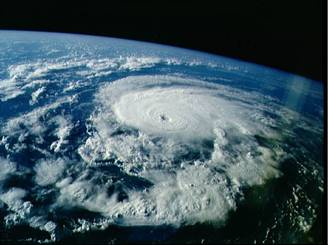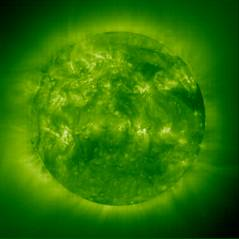Fluid Physics

12.330J/8.292J

Spring 2004

COURSE OUTLINE

Introduction: Outstanding problems in fluid physics

Definition of fluid; statistical mechanics

Hydrostatics, mass continuity

The Bernoulli Equation

The shallow-water equations

Gravity waves

Hydraulic jumps, bores

Surface waves

Potential flow

Kelvin’s circulation theorem

Forces on objects

Some real-world problems

Compressible flow

Thermodynamics

Sound waves

Shocks

Bernoulli Equation for compressible flows

Stratified flows

Internal waves

Flow over topography

Rotating flows

Barotropic flows, point vortices

Rossby waves

Baroclinic flows

Instabilities

Convection

Rayleigh shear flow instability

Kelvin-Helmholtz instability

Viscous flows

Stress

The Navier-Stokes equations

Viscous dissipation

Ekman layers

Turbulence

Physics of fluid phenomena

Stellar dynamics

Hurricanes

Superfluids

Other